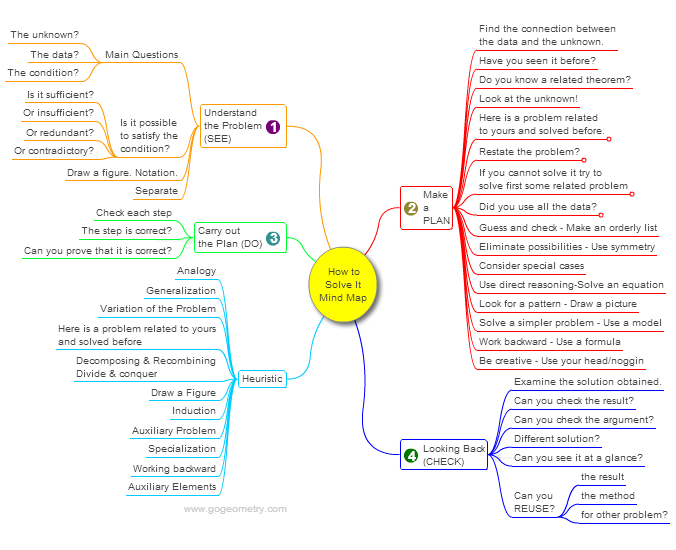< PREVIOUS PROBLEM  |  NEXT PROBLEM >

Problem 1014. Square, Diagonal, 45 Degrees, Angle, Equal Areas, Triangle, Quadrilateral
 In the figure below, ABCD is a square and the measure of angle EAF is 45 degrees (E on BC and F on CD). AE and AF intersect BD at G and H, respectively. Prove that the areas of triangle AGH and quadrilateral EFHG are equal.## Mind Map: George Pólya's 1945 book, "How to Solve It"

George Pólya's 1945 book "How to Solve It, A new aspect of Mathematical Method", is a book describing methods of problem solving. It suggests the following steps when solving a mathematical problem: (1) First, you have to understand the problem. (2) After understanding, then make a plan. (3) Carry out the plan. (4) Look back on your work. How could it be better?
A mind map is a diagram used to visually organize information, knowledge, concepts or ideas.Home | SearchGeometry | Problems | All Problems | Open Problems | Visual Index | 10 Problems | Problems Art GalleryArt | 1011-1020 | Triangle | Square | Quadrilaterals | 45 degreesAreas | Email | Solution / comment | by Antonio Gutierrez
Last updated: Sep 19, 2014